# GSEB Solutions Class 11 Maths Chapter 6 Linear Inequalities Ex 6.3

Gujarat Board GSEB Textbook Solutions Class 11 Maths Chapter 6 Linear Inequalities Ex 6.3 Textbook Questions and Answers.

## Gujarat Board Textbook Solutions Class 11 Maths Chapter 6 Linear Inequalities Ex 6.3

Solve the following systems of inequalities graphically:
1. x ≥ 3, y ≥ 2
2. 3x + 2y ≤ 12, x ≥ 1, y ≥ 2
3. 2x + y ≥ 6, 3x + 4y ≤ 12
4. x + y > 4, 2x – y > 0
5. 2x – y > 1, x – 2y < – 1
6. x + y ≤ 6, x + y ≥ 4
7. 2x + y ≥ 8, x + 2y ≥ 10
8. x + y ≤ 9, y > x, x ≥ 0
9. 5x + 4y ≤ 20, x ≥ 1, y ≥ 2
10. 3x + 4y < 60, x + 3y ≤ 30, x ≥ 30, x ≥ 0, y ≥ 0
11. 2x + y ≥ 4, x + y ≤ 3, 2x – y ≤ 6
12. x – 2y ≤ 3, 3x + 4y ≥ 12, x ≥ 0, y ≥ 1
13. 4x + 3y ≤ 60, y ≤ 2x, x ≥ 3, y ≥ 0
14. 3x + 2y ≤ 150, x + 4y ≤ 80, x ≤ 15, y > 0
15. x + 2y < 10, x + y ≥ 1, x – y ≤ 0, x ≥ 0, y ≥ 0
Solutions to questions 1 – 15:
1. x ≥ 3, y ≥ 2
(i) The line x = 3 passes through the points (3, 0), (3, 2). AB represents the line x = 3.
Putting x = 0 in x ≥ 3
0 ≥ 3 is not true.
∴ Origin does not lie in the region x ≥ 3.
Its graph is shaded with the horizontal lines.

(ii) The line y = 2 passes through the points (0, 2) and (2, 2).
CD represents the line y = 2.
Putting y = 0 in y ≥ 2
0 ≥ 2 is not true.
∴ Origin does not lie in the region y ≥ 2.
The graph of y ≥ 2, is shown by vertical lines.
Solution of x ≥ 3 and y ≥ 2 is the graph common to horizontal and vertical lines in PBQ.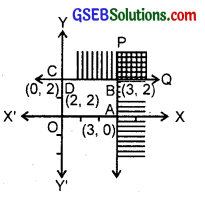2. Inequalities are 3x + 2y ≤ 12, x ≥ 1, y ≥ 2.
(i) The line 3x + 2y = 12 passes through (4, 0), (0, 6).
AB represents the line
3x + 2y = 12
Putting x = 0, y = 0 in
3x + 2y ≤ 12
0 + 0 ≤ 12, which is true.
∴ Origin lies in the region 3x + 2y ≤ 12.
i.e., 3x + 2y ≤ 12 represents the area below the line 3x + 2y = 12 and all the points lying on it.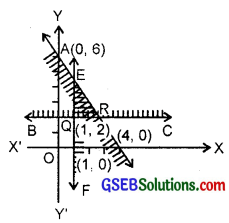(ii) The line x = 1 passes through (1, 0) and (1, 2). This line is represented by EF.
Consider the inequality x ≥ 1
Putting x = 0, 0 ≥ 1 is not true.
∴ Origin does not lie in the region x ≥ 1.
The region on the right of EF and the points on EF form the inequality x ≥ 1.

(iii) The line y = 2, passes through the points (1, 2), (2, 2). This line is represented by BC.
Putting y = 0 in y ≥ 2, we get 0 ≥ 2 which is not true.
∴ Origin does not lie in this region.
y ≥ 2 is represented by the region above y = 2 and all the points on this line.
The region satisfying the inequalities
3x + 2y ≤ 12, x ≥ 1, y ≥ 2 is the ∆ PQR.3. The inequalities are 2x + y ≥ 6,
3x + 4y ≤ 12
(i) The line 2x + y = 6 passes AB represents the equation 2x + y = 6.
Putting x = 0, y = 0 in 2x + y ≥ 6.
0 ≥ 6 which is not true.
∴ Origin does not lie in this region.∴ The region lying above AB and all the points on AB represent the inequality
2x + y ≥ 6

(ii) The line 3x + 4y = 12 passes through (4, 0) and (0, 3).
This is represented by CD.
Putting x = 0, y = 0 in 3x + 4y ≤ 12, we get 0 ≤ 12, which is true.
∴ 3x + 4y ≤ 12 represents the region below CD (towards origin) and all the points lying on it.
The common region is the solution of 2x + 3y ≥ 6 are 3x + 4y ≤ 12 represented by the area between 4, 2x – y > 0
(i) The line x + y = 4 passes through (4, 0) and (0, 4). This is represented by AB.
Putting x = 0, y = 0 sin x + y > 4
0 > 4 is false.
∴ Origin does not lie in the region.
or x + y > 4 is represented by the region above the line x + y = 4.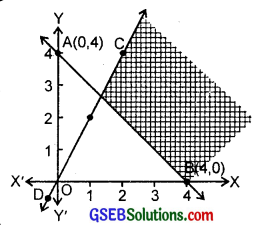(ii) The line 2x – y = 0
passes through (0, 0) and (1, 2).
This is represented by CD.
Putting x = 1, y = 0, 2 > 0 is true.
This shows (1, 0) lies in the region.
i.e., region lying below the line 2x – y = 0 represents 2x – y > 0.
The common region to both inequalities is the region contained in the <BPC, as shown in the figure. 5.
2x – y > 1 ……………….. (1)
and x – 2y < – 1 …………………. (2)
Graph of inequality (1): Let us draw the graph of line
2x – y = 1 ………………………. (3)
at y = 0, x = $$\frac{1}{2}$$, the point A is ($$\frac{1}{2}$$, 0). at x = 0, y = – 1, the point B is (0, – 1).Putting x = y = 0 in (1), we have 0 > 1, which is false.
Hence, half plane region II lying below AB is the solution region which is the shaded area (not including the line AB).

Graph of inequality (2):
Let us draw the graph of the line x – 2y = – 1 ……………….. (4)
Putting y = 0, x = – 1, point C is (- 1, 0).
Putting x = 0, y = $$\frac{1}{2}$$ point D is (0, $$\frac{1}{2}$$). Graph of (4) is the line CD in the figure.
Putting x = y = 0 in (2), we have 0 < – 1, which is false.
Hence, half plane region II lying above AB is the solution area. Double shaded area is the solution region of the given inequalities.6. The inequalities are x + y ≤ 6 and x + y ≥ 4.
(i) The line x + y = 6 passes through (6, 0) and (0, 6). It is represented by AB.
Putting x = 0, y = 0 in x + y ≤ 6
0 < 6 is true.
∴ Origin lies in the region x + y ≤ 6.
∴ x + y ≤ 6 is represented by the region below the line x + y = 6 and all the points lying on the line x + y = 6.(ii) The line x + y = 4 passes through (4, 0) and (0, 4).
It is represented by CD.
Putting x = 0, y = 0 in x + y ≥ 4 or 0 + 0 ≥ 4 is false.
∴ Origin does not lie in this region.
∴ The points lying above this line and the points lying on this line represent the inequality x + y ≥ 4.
The solution region is the origin lying between AB and CD and all the points lying on these lines.

7. 2x + y ≥ 8 ………………… (1)
and x + 2y ≥ 10 ……………… (2)
Graph of inequality (1):
Let us draw the graph of the line
2x + y = 8 …………………. (3)
at y = 0, x = 4
∴ Point A(4, 0) at x = 0, y = 8.
∴ Point B(0, 8).Putting x = y = 0 in (1), we have 0 ≥ 8, which is false.
Hence, half plane region II lying above AB and including the line AB is the area of solution.

Graph of the inequality (2):
Let us draw the graph of line x + 2y = 10.
At y = 0, x = 10.
Point C(10, 0).
Also, at x = 0, y = 5. So, point D is (0, 5).
Putting x = 0, y = 0 in (2), we have 0 ≥ 10, which is false.
Hence, upper portion of the line CD including the line CD is the shaded area. Double shaded area of figure is the solution region.8. The inequalities are x + y ≤ 9, y > x, x ≥ 0.
(i) The line x + y = 9 passes through (9, 0) and (0, 9).
AB represents this line.
Putting x = 0, y = 0 sin x + y ≤ 9
0 + 0 = 0 < 9, which is true. Origin lies in this region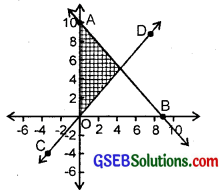∴ Region below the line AB and all the points on it form the region x + y ≤ 9.

(ii) The line y = x, passes through the origin and (2, 2). ∴ CD represents the line y = x. Putting x = 0, y = 1 sin y – x > 0
1 – 0 > 0 which is true.
∴ (0, 1) lies even in this region.
The inequality y > x is represented by the region above the line CD.

(iii) x > 0, (1, 0) lies in this region.
The region x > 0 lies on the right of y-axis.
Region bounded by ∆ PQR is the solution of + y ≤ 9, y > x, x > 0.

9. The inequalities are 5x + 4y ≤ 20, x ≥ 1, y ≥ 2.
(i) The line 5x + 4y = 20 passes through (4, 0) and (0, 5). This line is represented by AB.
Putting x = 0, y = 0
0 + 0 = 0 < 20, which is true.
The origin lies in this region, i.e., region below the line 5x + 4y = 20 and all the points lying on it belong to 5x + 4y ≤ 20.

(ii) y = 2 is the line parallel to x – axis at a distance 2 from the origin.
It is represented by EF. Putting y = 0, 0 ≥ 2 is not true.
Origin does not lie in this region.
Region above y = 2 represents the inequality y ≥ 2 including the points lying on it.

(iii) x = 1 is the line parallel to y-axis at a distance 1. from the origin.
Putting x = 0, in x – 1 ≥ 0, we get
– 1 ≥ 0, which is not true.
Origin does not lie in this region.
∴ The region on the right of x = 1 and all the points lying on it belong to x ≥ 1.
Double shaded region bounded by A PQR is the solution of given inequalities.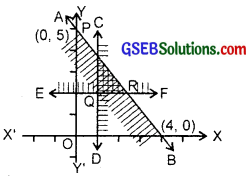10. The inequalities are
3x + 4y ≤ 60 …………….. (1)
x + 3y ≤ 30 ………………. (2)
x ≥ 0 …………….. (3)
y ≥ 0 ……………… (4)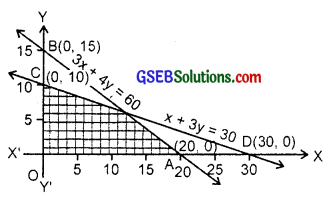We first draw the graphs of lines 3x + 4y = 60, x + 3y = 30, x = 0 and y = 0.
The line 3x + 4y = 60 passes through A (20, 0) and B(0, 15).
Since 0 < 60, origin lies in this region.
∴ 3x + 4y ≤ 60 represents the region below it and all the points on AB.
Further, x + 3y = 30 passes through (0, 10) and (30, 0).
CD represents this line.
∴ 0 < 30 is true. So, origin lies in the region x + 3y ≤ 30.
This inequality represent the region below it and the line itself.
Thus, we note that inequalities (1) and (2) represent the two shaded regions below the respective lines (including the lines) (see figure).
Inequality (3) represents the region on the right of y-axis and the y-axis is itself.
Inequality (4) represents the region above x-axis and the x-axis itself.
Multishaded region is the solution area.

11. 2x + y ≥ 4 ………………… (1)
x + y ≤ 3 ………………. (2)
2x – 3y ≤ 6 ……………….. (3)
We first draw the graphs of equations 2x + y = 4, x + y = 3 and 2x – 3y = 6.2x + y = 4, passes through A(2, 0) and B(0, 4).
Therefore, graph of it is AB.
Since 0 ≯ 4, so origin does not lie in region of 2x + y ≥ 4.
This inequality represents the region above it and all the points of the line 2x + y = 0.
Again x + y = 3 is represented by the line CD, where C is (3, 0) and D(0, 3).
As 0 < 3, origin lies in the region of x + y ≤ 3.
∴ x + y ≤ 3 represents the region below CD and all the points of CD.
Further, 2x – 3y = 6 is represented by EC, where E is (0, – 2) and C is (3, 0).
As 0 < 6, origin lies in it.
∴ 2x – 3y ≤ 6 represents the region below EC and all the points of EC.
Triple shaded triangular region is the solution area in the solution region.12. The inequalities are x – 2y ≤ 3, 3x + 4y ≥ 12, x ≥ 0, y ≥ 1.
(i) The line x – 2y = 3 passes through (3, 0) and (0, $$\frac{3}{2}$$)
This is represented by AB. 0 < 3 denotes origin lies in the region x – 2y ≤ 3.
∴ Region above this line and including its points represents x – 2y ≤ 3.

(ii) 3x + 4y = 12 passes through (4, 0) and (0, 3). CD represents this line.
Since 0 ≯ 12, so origin does not lies in 3x + 4y ≥ 12.
The region above CD and including points of CD represents 3x + 4y ≥ 12.

(iii) x ≥ 0 the region on the right of y-axis and all the points on it.

(iv) y = 1 is the line parallel to x-axis at a distance 1 from it. y ≥ 1 or y – 1 > 0.
Since – 1 ≯ 0, so origin does not lie in this region.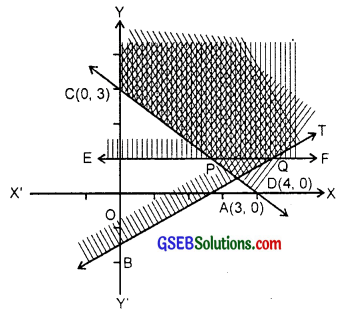∴ y ≥ 1 is the region above y = 1 and the points lying on it.
The common shaded region is YC PQT, which represents the solution of given inequalities.

13. The inequalities are 4x + 3y ≤ 60, y ≤ 2x, x ≥ 3, x, y ≥ 0
(i) The line 4x + 3y = 60 passes through (15, 0), (0, 20) and it is represented by AB.
Putting x = 0, y = 0, we get
in 3x + 4y ≤ 60, we get
0 + 0 = 0 ≤ 60 which is true. Therefore, origin lies in this region.
Thus, region below AB and the points lying on AB represent the inequality 4x + 3y ≤ 60.

(ii) y = 2x
passes through (0, 0) and (5, 10). It is represented by CD.
Putting x = 0, y = 5 in y – 2x ≤ 0, we get
5 < 0 is not true. ∴ (0, 5) does not lie in this region.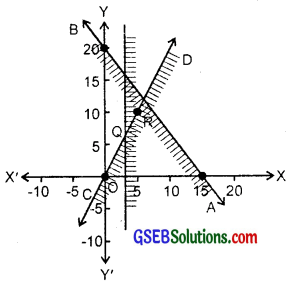∴ Region lying above the line CD and including the points on CD represents y ≥ 2x.

(iii) x ≥ 3 is the region lying on the right of line x = 3 and points lying on x = 3 represent the inequality x ≥ 3. The shaded area is the ∆ OAR in which x > 0 and y > 0 is true for each point, is the solution of given inequalities.14. The inequalities are 3x + 2y ≤ 150, x + 4y ≤ 80, x ≤ 15, y ≥ 0.
The line 3x + 2y = 150 passes through the points (50, 0) and (0, 75). AB represents this line.
Putting x = 0, y = 0 in 3x + 2y ≤ 150
⇒ 0 ≤ 150.
It shows that origin lies in this region.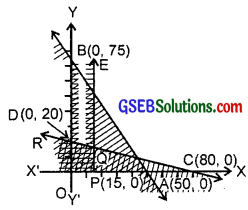The region lying below AB and the points lying on AB represent the inequality 3x + 2y ≤ 150.

(ii) The equation x + 4y = 80 passes through the points (80, 0), (0, 20).
This is represented by CD.
Since 0 < 80, so a region lying below CD and the points of CD represent the inequality x + 4y ≤ 80.

(iii) x ≤ 15 is the region lying on the left of EF where equation is x = 15 and the points lying on EF.
Thus the region X’PQR, which is a double shaded region, is the solution of the given inequalities. It may be noted that any point lying in this region satisfies the inequality 3x + 2y ≤ 150 also.

15. The inequalities are x + 2y ≤ 10, x + y ≥ 1, x – y ≤ 0, x ≥ 0, y ≥ 0.
(i) x + 2y = 10 passes through (10, 0) and (0, 5). The line AB represents this equation. Since 0 < 10, therefore origin lies in the region x + 2y ≤ 0.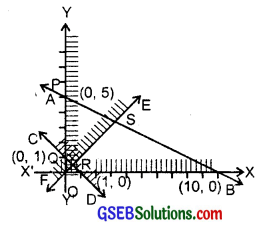∴ Region lying below AB and the points lying on it represents
x + 2y ≤ 10

(ii) x + y = 1 passes through (1, 0) and (0, 1). The line CD represents this equation. Since 0 ≯ 1, therefore origin does not lie on x + y ≥ 1.
∴ The region lying above the line CD and the points lying on CD represents the inequality x + y ≥ 1.

(iii) x – y = 0, passes through (0, 0) and (1, 1). This is being represented by EF. Also, (0, 1) lies on x – y ≤ 0.
The region lying above EF and the points lying on it represents the inequality x – y ≤ 0.

(iv) x ≥ 0 is the region lying on the right of y-axis and the points lying on y-axis.

(v) y ≥ 0 is the region above x-axis, and the points lying on y = 0.
The shaded region PQRS represents the given inequalities including y ≥ 0 since each point of region PQRS satisfied the condition y ≥ 0.